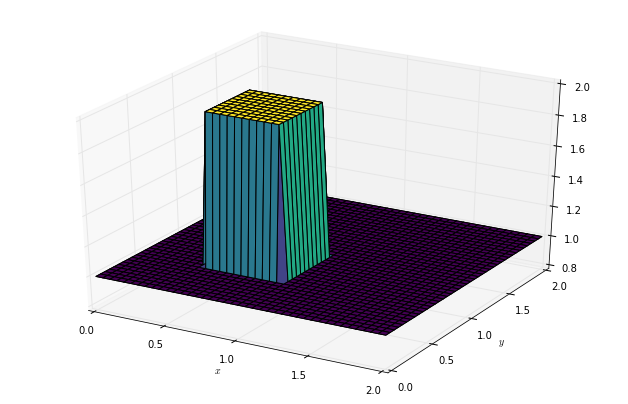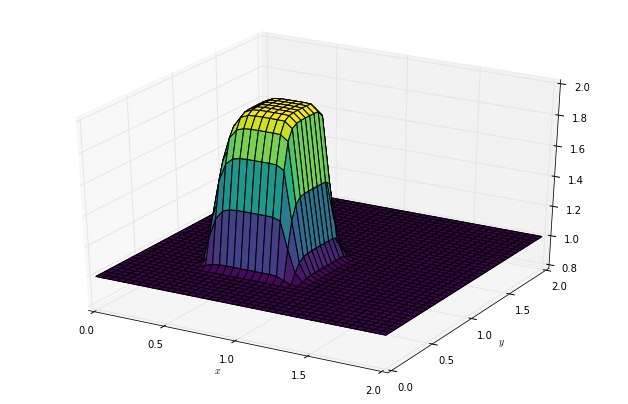Text provided under a Creative Commons Attribution license, CC-BY. All code is made available under the FSF-approved BSD-3 license. (c) Lorena A. Barba, Gilbert F. Forsyth 2017. Thanks to NSF for support via CAREER award #1149784.

# 12 steps to Navier–Stokes¶

This will be a milestone! We now get to Step 8: Burgers' equation. We can learn so much more from this equation. It plays a very important role in fluid mechanics, because it contains the full convective nonlinearity of the flow equations, and at the same time there are many known analytical solutions.

## Step 8: Burgers' Equation in 2D¶

Remember, Burgers' equation can generate discontinuous solutions from an initial condition that is smooth, i.e., can develop "shocks." We want to see this in two dimensions now!

Here is our coupled set of PDEs:

$$\frac{\partial u}{\partial t} + u \frac{\partial u}{\partial x} + v \frac{\partial u}{\partial y} = \nu \; \left(\frac{\partial ^2 u}{\partial x^2} + \frac{\partial ^2 u}{\partial y^2}\right)$$$$\frac{\partial v}{\partial t} + u \frac{\partial v}{\partial x} + v \frac{\partial v}{\partial y} = \nu \; \left(\frac{\partial ^2 v}{\partial x^2} + \frac{\partial ^2 v}{\partial y^2}\right)$$

We know how to discretize each term: we've already done it before!

$$\begin{split} & \frac{u_{i,j}^{n+1} - u_{i,j}^n}{\Delta t} + u_{i,j}^n \frac{u_{i,j}^n-u_{i-1,j}^n}{\Delta x} + v_{i,j}^n \frac{u_{i,j}^n - u_{i,j-1}^n}{\Delta y} = \\ & \qquad \nu \left( \frac{u_{i+1,j}^n - 2u_{i,j}^n+u_{i-1,j}^n}{\Delta x^2} + \frac{u_{i,j+1}^n - 2u_{i,j}^n + u_{i,j-1}^n}{\Delta y^2} \right) \end{split}$$
$$\begin{split} & \frac{v_{i,j}^{n+1} - v_{i,j}^n}{\Delta t} + u_{i,j}^n \frac{v_{i,j}^n-v_{i-1,j}^n}{\Delta x} + v_{i,j}^n \frac{v_{i,j}^n - v_{i,j-1}^n}{\Delta y} = \\ & \qquad \nu \left( \frac{v_{i+1,j}^n - 2v_{i,j}^n+v_{i-1,j}^n}{\Delta x^2} + \frac{v_{i,j+1}^n - 2v_{i,j}^n + v_{i,j-1}^n}{\Delta y^2} \right) \end{split}$$

And now, we will rearrange each of these equations for the only unknown: the two components $u,v$ of the solution at the next time step:

$$\begin{split} u_{i,j}^{n+1} = & u_{i,j}^n - \frac{\Delta t}{\Delta x} u_{i,j}^n (u_{i,j}^n - u_{i-1,j}^n) - \frac{\Delta t}{\Delta y} v_{i,j}^n (u_{i,j}^n - u_{i,j-1}^n) \\ &+ \frac{\nu \Delta t}{\Delta x^2}(u_{i+1,j}^n-2u_{i,j}^n+u_{i-1,j}^n) + \frac{\nu \Delta t}{\Delta y^2} (u_{i,j+1}^n - 2u_{i,j}^n + u_{i,j-1}^n) \end{split}$$
$$\begin{split} v_{i,j}^{n+1} = & v_{i,j}^n - \frac{\Delta t}{\Delta x} u_{i,j}^n (v_{i,j}^n - v_{i-1,j}^n) - \frac{\Delta t}{\Delta y} v_{i,j}^n (v_{i,j}^n - v_{i,j-1}^n) \\ &+ \frac{\nu \Delta t}{\Delta x^2}(v_{i+1,j}^n-2v_{i,j}^n+v_{i-1,j}^n) + \frac{\nu \Delta t}{\Delta y^2} (v_{i,j+1}^n - 2v_{i,j}^n + v_{i,j-1}^n) \end{split}$$
In :
import numpy
from matplotlib import pyplot, cm
from mpl_toolkits.mplot3d import Axes3D
%matplotlib inline

In :
###variable declarations
nx = 41
ny = 41
nt = 120
c = 1
dx = 2 / (nx - 1)
dy = 2 / (ny - 1)
sigma = .0009
nu = 0.01
dt = sigma * dx * dy / nu

x = numpy.linspace(0, 2, nx)
y = numpy.linspace(0, 2, ny)

u = numpy.ones((ny, nx))  # create a 1xn vector of 1's
v = numpy.ones((ny, nx))
un = numpy.ones((ny, nx))
vn = numpy.ones((ny, nx))
comb = numpy.ones((ny, nx))

##set hat function I.C. : u(.5<=x<=1 && .5<=y<=1 ) is 2
u[int(.5 / dy):int(1 / dy + 1),int(.5 / dx):int(1 / dx + 1)] = 2
##set hat function I.C. : u(.5<=x<=1 && .5<=y<=1 ) is 2
v[int(.5 / dy):int(1 / dy + 1),int(.5 / dx):int(1 / dx + 1)] = 2

In :
###(plot ICs)
fig = pyplot.figure(figsize=(11, 7), dpi=100)
ax = fig.gca(projection='3d')
X, Y = numpy.meshgrid(x, y)
ax.plot_surface(X, Y, u[:], cmap=cm.viridis, rstride=1, cstride=1)
ax.plot_surface(X, Y, v[:], cmap=cm.viridis, rstride=1, cstride=1)
ax.set_xlabel('$x$')
ax.set_ylabel('$y$');In :
for n in range(nt + 1): ##loop across number of time steps
un = u.copy()
vn = v.copy()

u[1:-1, 1:-1] = (un[1:-1, 1:-1] -
dt / dx * un[1:-1, 1:-1] *
(un[1:-1, 1:-1] - un[1:-1, 0:-2]) -
dt / dy * vn[1:-1, 1:-1] *
(un[1:-1, 1:-1] - un[0:-2, 1:-1]) +
nu * dt / dx**2 *
(un[1:-1,2:] - 2 * un[1:-1, 1:-1] + un[1:-1, 0:-2]) +
nu * dt / dy**2 *
(un[2:, 1:-1] - 2 * un[1:-1, 1:-1] + un[0:-2, 1:-1]))

v[1:-1, 1:-1] = (vn[1:-1, 1:-1] -
dt / dx * un[1:-1, 1:-1] *
(vn[1:-1, 1:-1] - vn[1:-1, 0:-2]) -
dt / dy * vn[1:-1, 1:-1] *
(vn[1:-1, 1:-1] - vn[0:-2, 1:-1]) +
nu * dt / dx**2 *
(vn[1:-1, 2:] - 2 * vn[1:-1, 1:-1] + vn[1:-1, 0:-2]) +
nu * dt / dy**2 *
(vn[2:, 1:-1] - 2 * vn[1:-1, 1:-1] + vn[0:-2, 1:-1]))

u[0, :] = 1
u[-1, :] = 1
u[:, 0] = 1
u[:, -1] = 1

v[0, :] = 1
v[-1, :] = 1
v[:, 0] = 1
v[:, -1] = 1

In :
fig = pyplot.figure(figsize=(11, 7), dpi=100)
ax = fig.gca(projection='3d')
X, Y = numpy.meshgrid(x, y)
ax.plot_surface(X, Y, u, cmap=cm.viridis, rstride=1, cstride=1)
ax.plot_surface(X, Y, v, cmap=cm.viridis, rstride=1, cstride=1)
ax.set_xlabel('$x$')
ax.set_ylabel('$y$');The video lesson that walks you through the details for Steps 5 to 8 is Video Lesson 6 on You Tube:

In :
from IPython.display import YouTubeVideo

from IPython.core.display import HTML